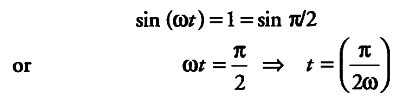# The position x of a body is given by x = A sin (cot)

The position x of a body is given by x = A sin (ωt). Find the time at which the displacement is maximum.

The value of position x will be maximum, when the value of sin (ωt) is maximum, for this